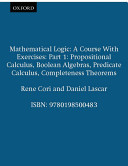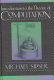### Mathematical Logic and Computability

Data is displayed for academic year: 2023./2024.

#### Course Description

The course is divided into three parts. In the first part we consider propositional logic. The main aim of the first part is to emphasize the difference between syntax and semantics. The first part is a motivation and introduction for the second part in that we study first-order logic. Specially considered is the undecidability of first-order logic, and a necessity of a formal definition of algorithm is emphasized. In the third part we study theory of computation. RAM-machines are considered as a basic model of computation. Then we define the class of partial recursive function, and prove that the class of partial recursive functions is equal to the class of RAM-computable functions. It is a motivation for Church thesis.

#### Study Programmes

[FER3-HR] Audio Technologies and Electroacoustics - profile
Elective Courses (1. semester) (3. semester)
[FER3-HR] Communication and Space Technologies - profile
Elective Courses (1. semester) (3. semester)
[FER3-HR] Computational Modelling in Engineering - profile
Elective Courses (1. semester) (3. semester)
[FER3-HR] Computer Engineering - profile
Elective Courses (1. semester) (3. semester)
[FER3-HR] Computer Science - profile
Elective Courses (1. semester) (3. semester)
[FER3-HR] Control Systems and Robotics - profile
Elective Courses (1. semester) (3. semester)
[FER3-HR] Data Science - profile
Elective Courses (1. semester) (3. semester)
[FER3-HR] Electrical Power Engineering - profile
Elective Courses (1. semester) (3. semester)
[FER3-HR] Electric Machines, Drives and Automation - profile
Elective Courses (1. semester) (3. semester)
[FER3-HR] Electronic and Computer Engineering - profile
Elective Courses (1. semester) (3. semester)
[FER3-HR] Electronics - profile
Elective Courses (1. semester) (3. semester)
[FER3-HR] Information and Communication Engineering - profile
Elective Courses (1. semester) (3. semester)
[FER3-HR] Network Science - profile
Elective Courses (1. semester) (3. semester)
[FER3-HR] Software Engineering and Information Systems - profile
Elective Course of the Profile (1. semester)
Elective Courses (1. semester)
[FER2-HR] Computer Engineering - profile
Mathematics and Science (1. semester)
[FER2-HR] Computer Science - profile
Mathematics and Science (1. semester)
[FER2-HR] Control Engineering and Automation - profile
Mathematics and Science (1. semester)
[FER2-HR] Electrical Engineering Systems and Technologies - profile
Mathematics and Science (1. semester)
[FER2-HR] Electrical Power Engineering - profile
Mathematics and Science (1. semester)
[FER2-HR] Electronic and Computer Engineering - profile
Mathematics and Science (1. semester)
[FER2-HR] Electronics - profile
Mathematics and Science (1. semester)
[FER2-HR] Information Processing - profile
Mathematics and Science (1. semester)
[FER2-HR] Software Engineering and Information Systems - profile
Mathematics and Science (1. semester)
[FER2-HR] Telecommunication and Informatics - profile
Mathematics and Science (1. semester)
[FER2-HR] Wireless Technologies - profile
Mathematics and Science (1. semester)

#### Learning Outcomes

1. convert a formula of propositional logic to normal form
2. define a notion of proof and theorem
3. practice semantic tableux
4. reproduce the basic facts on modal logic
5. define a notion of first-order structure
6. convert a first-order formula to prenex normal form
7. define the notions of RAM-computable and partial recursive functions
8. classify the import of Church thesis

#### Forms of Teaching

Lectures

Lectures which contain large number of examples and problems.

Continuous Assessment Exam
Homeworks 0 % 10 % 0 % 10 %
Mid Term Exam: Written 0 % 45 % 0 %
Final Exam: Written 0 % 45 %
Exam: Written 0 % 90 %

#### Week by Week Schedule

1. The basic on set theory. Introduction to propositional logic. Syntax of propositional logic. Semantics of propositional logic.
2. Normal forms in propositional logic. Semantic tableux. Decidability. Problem P=NP.
3. Axiomatic system for propositional logic. Proof, theorem and deduction. Theorem of adequacy. Consistency.
4. A sketch of proof of completeness theorem for propositional logic.
5. Modal logic: system K, Kripke frame and model, theorems on completeness and adequacy of system K.
6. First-order logic: syntax of a first-order theory, terms and formulas.
7. Semantics of first order logic: interpretation, truth of formulas. Prenex normal form.
8. Midterm exam
9. Semantic tableux for first-order logic. Church theorem.
10. Axiomatic system for first-order logic. Proof, theorem and deduction. Theorem of deduction. Theorem od adequacy.
11. Consistency. A sketch of proof of Goedel completeness theorem. Compactness theorem.
12. Computability: RAM-machine, RAM-computable function. Macro-machine.
13. Primitive recursive functions: initial functions, composition, primitive recursion. Ackermann function. Partial recursive function. Recursive sets.
14. Proof that each partial recursive function is RAM-computable. Coding of finite sequence of natural numbers. Coding of RAM-machine. Universal function.
15. Kleene normal form theorem on partial recursive function. The equality of classes of RAM-computable function and partial recursive functions. Recursion theorem. Rice theorem. Church thesis. Halting problem. A sketch oof proof of Church theorem.

#### Literature

Mladen Vuković (2009.), Matematička logika, Element, ZagrebR. Cori, D. Lascar (2000.), Mathematical Logic, Part I,, Oxford University PressMichael Sipser (1997.), Introduction to the Theory of Computation, Course Technology Ptr
J. R. Shoefield (2017.), Recursion Theory, Cambridge University Press

#### General

ID 222646
Winter semester
5 ECTS
L1 English Level
L1 e-Learning
45 Lectures
0 Seminar
0 Exercises
0 Laboratory exercises
0 Project laboratory